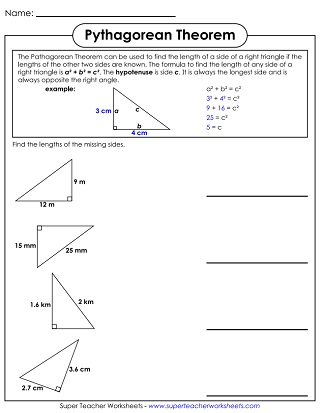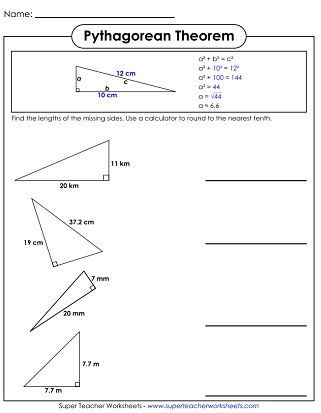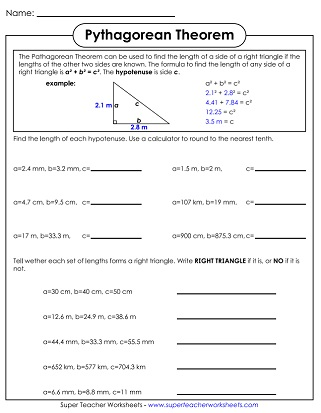# Pythagorean Theorem Worksheets

This page has printables for teaching students about the Pythagorean Theorem. On these sheets, students will be given right angles. They'll find the lengths of unknown sides, or the hypotenuse.## Level: Basic

Students must identify which side of a right triangle is the hypotenuse. Includes two word problems.
A worksheet where students use the Pythagorean Theorem to find the length of the missing side of right triangles. All triangle measurements are whole numbers and use Pythagorean triples.
This worksheet contains a set of numbers that students must use the Pythagorean Theorem to find the missing length of a right triangle as well as determine if each set of lengths forms a right triangle.

Like the Basic version, students use the Pythagorean Theorem to find the length of the missing side of right triangles. Decimals are used and a calculator is required to find square roots and rounding.
Another worksheet like the first with more difficult numbers. Students must both find the hypotenuse of a set of numbers, as well as figure out if a set of three numbers makes a right triangle.
Middle School Math Worksheets

Area Worksheets

Calculate the areas of rectangles, triangles, trapezoids, circles, and parallelograms.

## Worksheet ImagesMy Account
Site Information